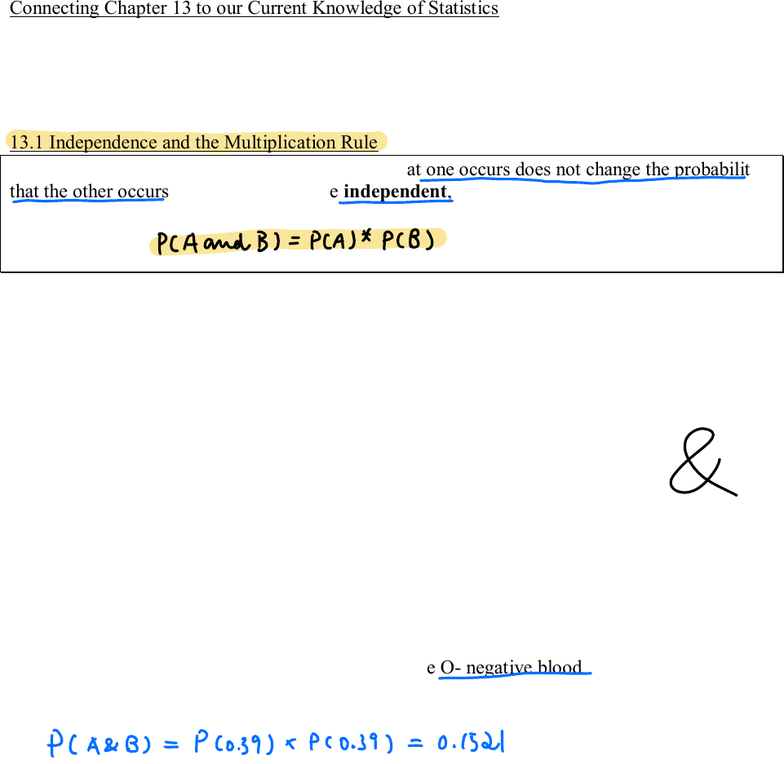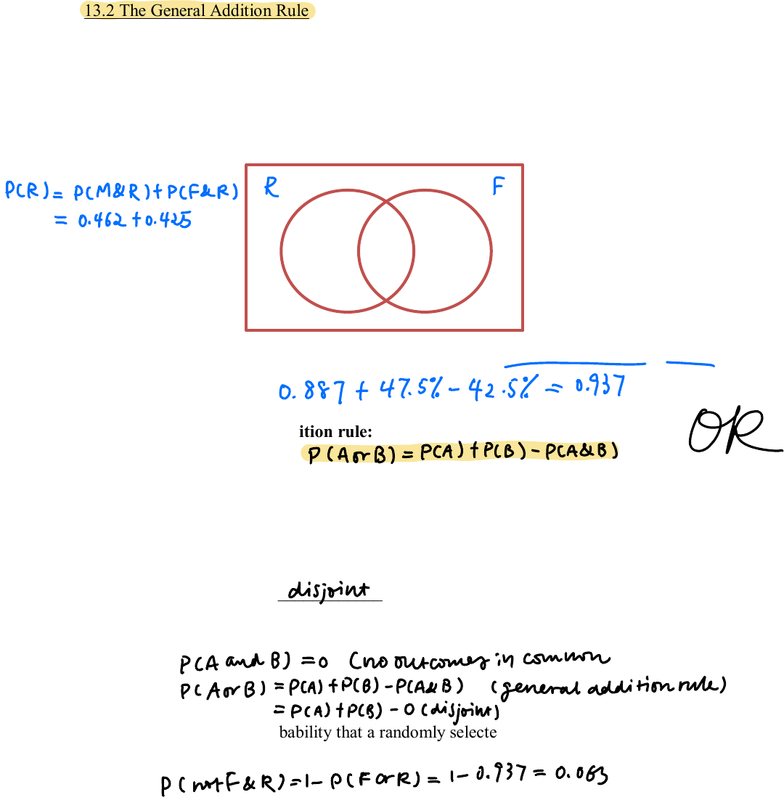# STAT 1450 Lecture Notes - Lecture 13: Probability Theory, Venn Diagram

20 views6 pages
School
Ohio State University
Department
Statistics
Course
STAT 1450
ProfessorChapter 13, page 1
STAT 1450 COURSE NOTES CHAPTER 13
GENERAL RULES OF PROBABILITY
Connecting Chapter 13 to our Current Knowledge of Statistics
Probability theory leads us from data collection to inference.
The introduction to probability from Chapter 12 will now be fortified by additional rules to allow
us to consider multiple types of events. The rules of probability will allow us to develop models
so that we can generalize from our (properly collected) sample to our population of interest.
13.1 Independence and the Multiplication Rule
Two events A and B are independent if knowing that one occurs does not change the probability
that the other occurs. Thus, if A and B are independent,
Example: Someone with type O-negative blood is considered to be a “universal donor.
According to the American Association of Blood Banks, 39% of people are type O-negative.
Two unrelated people are selected at random. Calculate the probability that both have type
O-negative blood.
Poll: Suppose two unrelated people are selected at random.
Calculate the probability that neither have type O- negative blood
(round to 4 decimal places).
PCAandBPCA PCB
PCABPco
39 xPC
0.39 0.1521
Unlock document

This preview shows pages 1-2 of the document.
Unlock all 6 pages and 3 million more documents.Chapter 13, page 2
Two-Way tables are helpful ways to picture two events.
Venn diagrams are an alternative means of displaying multiple events.
Both can be used to answer many questions involving probabilities.
Example: In a sample of 1000 people, 88.7% of them were right-hand dominant, 47.5% of them
were female, and 42.5% of them were female and right-hand dominant. Draw a Venn diagram
for this situation.
Calculate the probability that a randomly selected person is right-hand dominant or female.
We just used the general addition rule:
For any two events A and B,
Question: Where did we see this concept previously?
What if the two events A and B do not overlap?
Events A and B are ________________ if they have no outcomes in common.
Question: What is P(A or B) when A and B are disjoint?
Example: Calculate the probability that a randomly selected person is neither right-hand
dominant nor female.
We just used the complement rule: For any event A, P(A does not occur) = P(not A) = 1 P(A).
0.462
0.063
0.425
0.050
PCR PcM RHPCFdR RF
0.46210.425
0.8871 47.5 42.5 0.937
disjoint
PCAandBonooutcomesin common
PCAorB _PCA fPCB PCA Bgeneral
rule
pA1
PCB OC
disjoint
PChotF RlPCForR l0.937 0.063
Unlock document

This preview shows pages 1-2 of the document.
Unlock all 6 pages and 3 million more documents.

## Document Summary

Connecting chapter 13 to our current knowledge of statistics. Probability theory leads us from data collection to inference. The introduction to probability from chapter 12 will now be fortified by additional rules to allow us to consider multiple types of events. The rules of probability will allow us to develop models so that we can generalize from our (properly collected) sample to our population of interest. Two events a and b are independent if knowing that one occurs does not change the probability that the other occurs. Example: someone with type o-negative blood is considered to be a universal donor. (cid:180) According to the american association of blood banks, 39% of people are type o-negative. Poll: suppose two unrelated people are selected at random. Calculate the probability that neither have type o- negative blood (round to 4 decimal places). Two-way tables are helpful ways to picture two events. Venn diagrams are an alternative means of displaying multiple events.